You are using an outdated browser. Please upgrade your browser to improve your experience.## Ohms Law Calc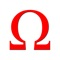# Ohms Law Calc.

by V PUGAZHENTHI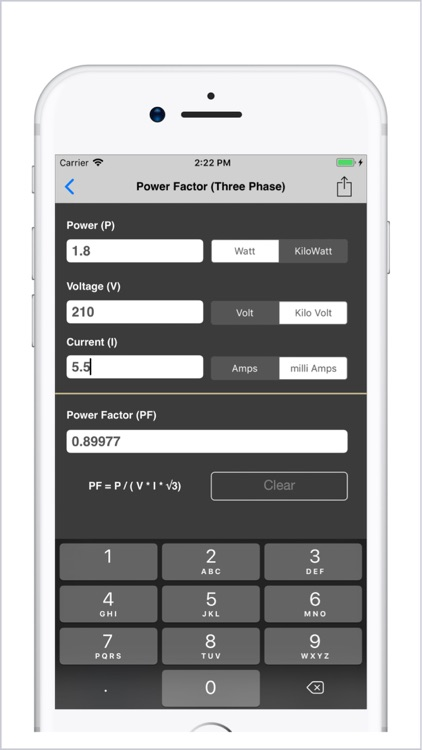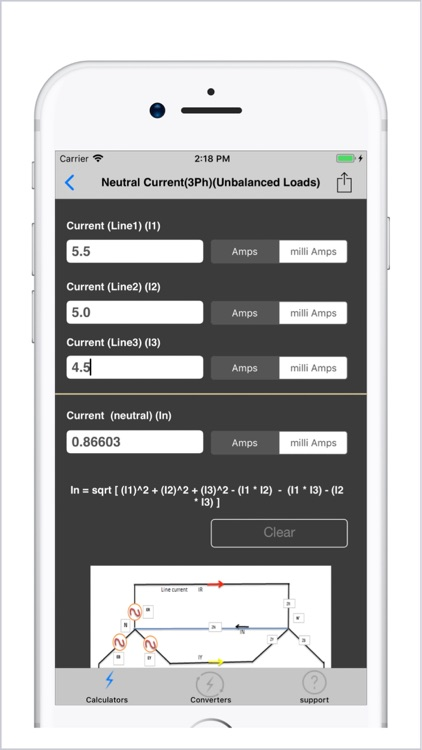### What is it about?

Ohms Law Calc. consists of### App Details

Version
8.2
Rating
(243)
Size
14Mb
Genre
Utilities Education
Last updated
March 14, 2019
Release date
January 6, 2012

### Ohms Law Calc is FREE but there are more add-ons

• \$3.99

Unlock all the 49 Calculators.

• \$0.99

16 - Electrical Converters

### App Screenshots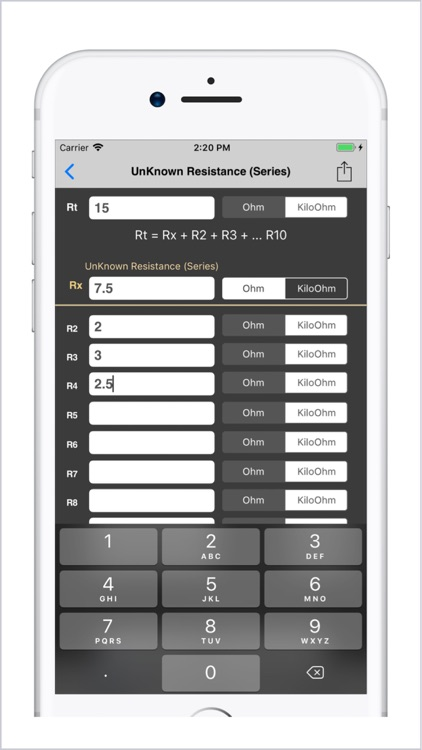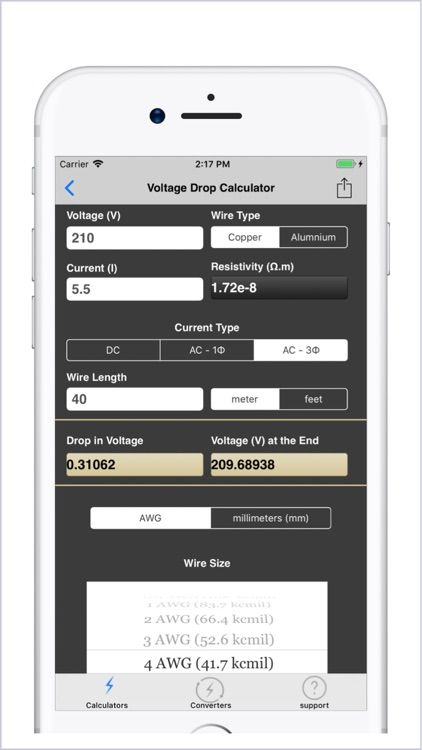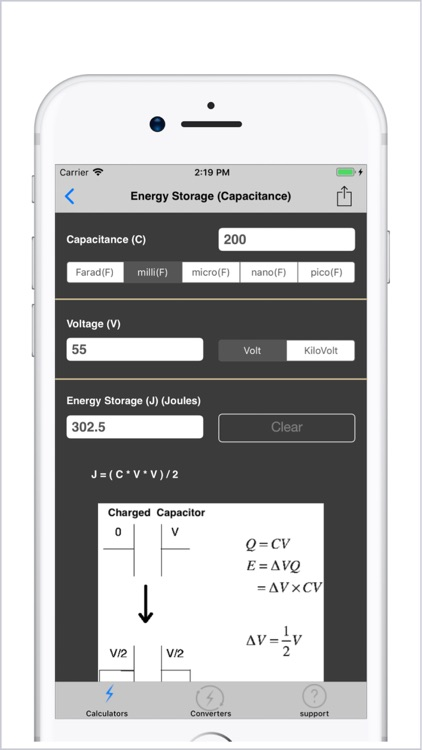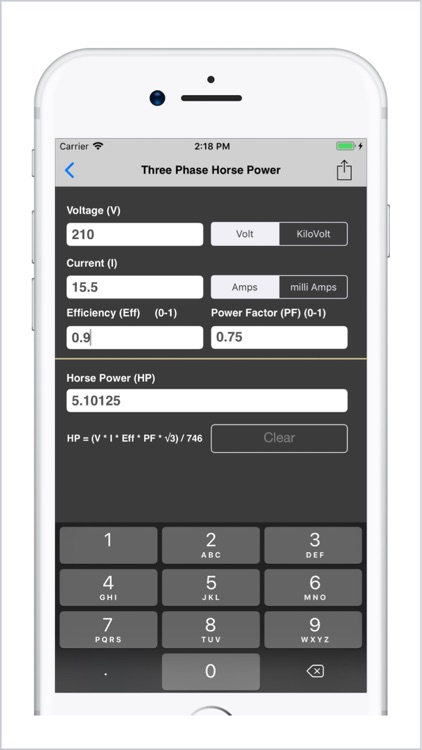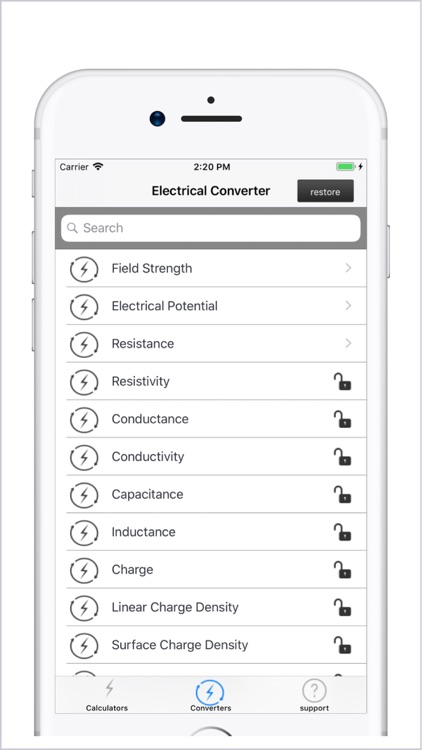### App Store Description

Ohms Law Calc. consists of
1.Voltage Calculator
2.Current Calculator
3.Resistance Calculator
4.Power Calculator
5.kVA to amps (Single Phase)
with Formula & Multiple Units.
More Calculators available for in-App Purchase :
- Voltage Drop Calculator( SI & USCS Standards for wire size )
6.kVA to amps (Three Phase)
7.amps to kVA (Single Phase)
8.amps to kVA (Three Phase)
9.Single Phase Power Calculator
10.Three Phase Power Calculator
11.Single Phase Current Calculator
12.Three Phase Current Calculator
13.DC Horse Power
14.Single Phase Horse Power
15.Three Phase Horse Power
16.DC Current (HP)Calculator
17.Single Phase Current (HP) Calculator
18.Three Phase Current (HP) Calculator
19.Efficiency (DC) Calculator
20.Efficiency (Single Phase) Calculator
21.Efficiency (Three Phase) Calculator
22.Power Factor (Single Phase) Calculator
23.Power Factor (Three Phase) Calculator
24.Luminous Intensity Calculator
25.Luminous Flux Calculator
26.Solid Angle Calculator
27.Energy Cost Calculator
28.Energy Storage (Resistance) Calculator
29.Energy Storage (Inductance) Calculator
30.Energy Storage (Capacitance) Calculator
31.Star to Delta Conversion
32.Delta to Star Conversion
33.Inductive Reactance Calculator
34.Capacitive Reactance Calculator
35.Resonant Frequency Calculator
36.Inductor Sizing Equation
37.Capacitor Sizing Equation
38.Resistance (Series) Calculator
39.Resistance (Parallel) Calculator
40.Inductance (Series) Calculator
41.Inductance (Parallel) Calculator
42.Capacitance (Series) Calculator
43.Capacitance (Parallel) Calculator
44.UnKnown Resistance (Series) Calculator
45.UnKnown Resistance (Parallel) Calculator
46.UnKnown Capacitance (Series) Calculator
47.UnKnown Capacitance (Parallel) Calculator
48.Neutral Current (3Ph) Unbalanced Loads

a.Change unit from amps to milliAmps or vice versa, the output Voltage is calculated instantly
b.Changes can be made to input units
c.Changes can be made to output units.

Disclaimer:
AppAdvice does not own this application and only provides images and links contained in the iTunes Search API, to help our users find the best apps to download. If you are the developer of this app and would like your information removed, please send a request to [email protected] and your information will be removed.